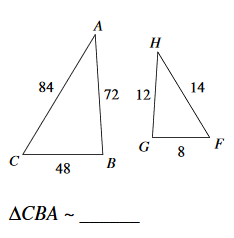Scale Factor
3 years ago
mssmith_north
Save
Edit
Host a game
Live GameLive
Homework
Solo Practice
Practice10 QuestionsShow answers
• Question 1
300 secondsQ. What is the scale factor?
4
.25
5
6
• Question 2
30 secondsQ. What is the measurement of x?
21
1.25
20
4
• Question 3
300 secondsQ. What is the measurement of x?
20 m
24 m
16 m
4 m
• Question 4
180 secondsQ. What is the scale factor?
2
3/2
2/3
1/2
• Question 5
120 secondsQ. What is the scale factor?
1/2
7/3
2
10/3
• Question 6
120 secondsQ. Solve the proportion.
____ = ____
p=31.3
p=308
p=924
p=532
• Question 7
900 secondsQ. Bobby has a piece of wood that has a height of 9, and a length of 4. If you have a different piece of wood that has a length of 16, what is the height?
6
4
12
9
• Question 8
300 seconds
Q. All corresponding angles are congruent in similar figures.
True
False
• Question 9
900 secondsQ. Rodney has a class project that needs to have a height of 10, and a length of 4. If he makes a scale model of a space ship. What would the height be if the length of the ship was 16?
60
40
12
120
• Question 10
900 secondsQ. Hilly and Amara are comparing drawings, Amara said her drawing was 20in tall and the length of 14in. What is the height of Billys drawing if it had a length of 7in?